﻿ Prediction of Ultimate Shear Capacity of Reinforced Normal and High Strength Concrete Beams Without Stirrups Using Fuzzy Logic

### Prediction of Ultimate Shear Capacity of Reinforced Normal and High Strength Concrete Beams Without ...

Abdulkhaliq Abdulyimah JaaferOPEN ACCESSPEER-REVIEWED

## Prediction of Ultimate Shear Capacity of Reinforced Normal and High Strength Concrete Beams Without Stirrups Using Fuzzy Logic

Abdulkhaliq Abdulyimah JaaferCivil Engineering Department, College of Engineering, Misan University, Misan, Iraq

### Abstract

The main objective of the present study is to predict the ultimate shear capacity of reinforced concrete beams no contains web reinforcement. Fuzzy inference system (FIS) was developed to predict the shear strength of these beams using Mamadani method. Fuzzy inference system (FIS) model has been proved to be very effective in predicting the ultimate shear strength of concrete beams without stirrups. The regression analysis between the output of the FIS model and the corresponding target, R2 = 0.9969 and 0.9509 for training and testing data, respectively. Based on FIS results, a parametric analysis was carried out to study the influence of each parameter affecting the shear strength of beams without stirrups and these results are compared with the provisions of ACI-code.

### At a glance: Figures

123456
Prev Next

• Jaafer, Abdulkhaliq Abdulyimah. "Prediction of Ultimate Shear Capacity of Reinforced Normal and High Strength Concrete Beams Without Stirrups Using Fuzzy Logic." American Journal of Civil Engineering and Architecture 1.4 (2013): 75-81.
• Jaafer, A. A. (2013). Prediction of Ultimate Shear Capacity of Reinforced Normal and High Strength Concrete Beams Without Stirrups Using Fuzzy Logic. American Journal of Civil Engineering and Architecture, 1(4), 75-81.
• Jaafer, Abdulkhaliq Abdulyimah. "Prediction of Ultimate Shear Capacity of Reinforced Normal and High Strength Concrete Beams Without Stirrups Using Fuzzy Logic." American Journal of Civil Engineering and Architecture 1, no. 4 (2013): 75-81.

 Import into BibTeX Import into EndNote Import into RefMan Import into RefWorks

### 1. Introduction

High-strength concrete (HSC) has been increasingly used in the construction industry during the last years. An increase in the strength of concrete is directly associated with an improvement in most of its properties, in particular the durability, but this also produces an increase in its brittleness and smoother crack surfaces which affects significantly the shear strength. The failure of HSC beams without web reinforcement occurs suddenly, and some current codes limit the magnitude of compressive strength used in the design formulae to around 60 MPa or 70 MPa to prevent it . Many researchers were studied the influence of different parameters that affecting the shear strength of beams. Collins and Kuchma  found out that HSC beam specimens showed a more significant size effect in shear than normal-strength concrete members. Experimental tests carried out by Fujita et al.  showed that shear fracture in HSC is characterised by a conspicuous localisation of cracking in comparison with ordinary strength concrete, and that the propagation of these cracks was rapid, resulting in a more brittle fracture.

In today fast paced world of increasing and innovative new technology, fuzzy logic is a practical mathematical addition to classic Boolean logic. Fuzzy logic is considered as a superset of standard logic which is extended to deal with partial truth. Fuzzy set theory is basically used to mathematically represent uncertainty and vagueness and provide tools to deal with the imprecision in many problems . For the last two decades, fuzzy set theory has been successfully applied in many different areas of engineering including automatic control, system identification, pattern recognition, design of structures, structural modeling and many more. There have been quite a good number of applications of fuzzy logic in different fields of civil engineering [5-11].

In this paper, a fuzzy inference system is developed for predicting ultimate shear strength of concrete rectangular beams without web reinforcement based on a database with 268 test beams and the results obtained are compared with those determined according to the ACI code for rectangular concrete beams.

### 2. ACI-Code Models

ACI code of practice presents two different procedures for calculating the failure shear strength for concrete beams without transverse reinforcement. The simplified method, equation 11-3, is as follows :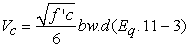(1)

The second procedure, equation 11-5, applies for those members, a/d≥1.4.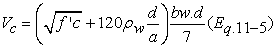(2)

### 3. Fuzzy Inference System (FIS)

3.1. General

Term "Fuzzy" was used by Prof. Lotfi Zadeh for the first time in 1962 . The "Fuzzy set" theory developed by Zadeh in 1965  provides as a mathematical framework to deal vagueness associated with the description of a variable. The fuzzy sets have since spread practically to all aspects of scientific disciplines. It may be regarded both as a generalization of classical set theory and as a generalization of dual logic.

The fuzzy inference system (FIS), also known as fuzzy rule-based systems or fuzzy models, is the process of formulating the mapping from a given input to an output using fuzzy logic. The dynamic behavior of an FIS is characterized by a set of linguistic description rules based on expert knowledge. This expert knowledge is usually of the form:

IF - a set of antecedent conditions is satisfied.

THEN - a set of consequences can be inferred.

3.2. Basic Design of FIS

In order to solve a problem which is based on uncertain or fuzzy observations or correlations, it is necessary to describe, map, and process the influencing factors in fuzzy terms and to provide the result of this processing in a useable form. These requirements result in the following basic elements of a FIS :

1 Knowledge base (definition of the linguistic variables, terms and rules)

2 Processing of the input variables (fuzzification)

3 Inference engine (analysis)

4 Processing results (defuzzification)

The (scalar) inputs are transformed into memberships of fuzzy sets by fuzzification functions. This information, together with the declared rules, is given to the inference engine; the result again being a set of memberships of fuzzy sets (terms for the output variables). The last step is to transform these membership values into the required scalar output variables by defuzzification. The following sections will discuss in detail each of these individual steps.

The knowledge base defines the relationships between the input and output parameters of a system. The most commonly used representation of the input-output relationships is Mamdani type fuzzy models. In this type of fuzzy models, linguistic propositions are used both in antecedent and consequent parts of the IF-THEN rules. Another type of representing the input-output relationships is Sugeno fuzzy models in which the antecedent part of the rules is composed of linguistic propositions, but the consequent parts is defined by either a constant number (zero order) or linear equations (first order). A Mamdani model and a zero-order Sugeno model of a multi-input-single-output system may be represented by a set of linear subsystems (rules) shown in Equation. (3) and Equation (4) respectively, as: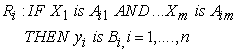(3)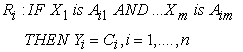(4)

Thus, every rule is a local fuzzy relationship that maps a part of the multidimensional input space U into a certain part of the output space V. The inference mechanism of Mamdani type, as shown in Figure (1a), consists of three connectives: the aggregation of antecedents in each rule (AND connectives), implication (i.e., IF-THEN connectives), and accumulation (or aggregation) of the rules (ALSO connectives). The operators performing the connectives distinguish the type of fuzzy inferencing. The AND and ALSO connectives are chosen from a family of t-norm (e.g., minimum and product operators) and t-conorm operators (e.g. maximum and sum operators), respectively. The implication (IF-THEN connective) also uses t-norm operators, but not necessarily identical to the ones used for the AND connectives.

The inference mechanism of Sugeno models (Figure (1b)) is more straightforward than the more common Mamdani’s type because the outputs of individual subsystems are crisp numbers. An algebraic product operator is usually selected to perform the t-norm to simplify the computations. The result of implication of each rule is a weight factor that indicates the rule degree of firing. The aggregation of the rules is simply adding the weighted result of the output of the individual rules.

### 4. FIS Model Development for Prediction Ultimate Shear Strength

The FIS is used to predict the ultimate shear strength of concrete beams. The FIS model is implemented using Fuzzy Logic Toolbox in MATLAB program version 7 (R14) . This program implements two different FIS models, Mamdani and Sugeno model. In this study, the results of using Mamadani FIS model is presented and discussed to examine the ability of this model to predict the ultimate shear strength of concrete beams without stirrups.

4.1. Preparation of Data

To provide sufficient information to train and verify the FIS, a comprehensive set of data has to be collected. An extensive review of the literature was therefore conducted to compile a database of test results on RC beams that fail in shear. All together, 268 test results available in literature were used . The data used to build the FIS model should be divided into two subsets: training set and validating or testing set. Among the collected data, 236 experimental data were sampled randomly and used for the training data (constructing rules) and the remaining 32 data for the testing data of the FIS model.

4.2. Input and Output Variables

The input and output variables are usually determined by the nature of the problem. In this study the parameters which may be introduced as the components of the input vector consist of the total depth to width ratio (d/bw), depth of beam (d), the concrete cylinder compressive strength (f'c), longitudinal reinforcement ratio (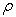) and shear span to depth ratio,(a/d). The output includes one output variable represents the ultimate shear force V (kN). The ranges of input and output variables are summarized in Table 1.

#### Table 1. Range of input and output parameters

4.3. Membership Functions

According to the collected data, and using the scatter method for partitioning, different linguistic terms to describe the input and output variables were chosen as shown in Table 2. To account for the non-linearity, each input variable is modeled using a Gaussian type membership function. While the output variable is modeled using a triangular (linear) type membership function. Based on this concept of the data classification, membership functions were determined for all input variables and output variable, as shown in Figures 2 to 7.

#### Table 2. Linguistic terms of input and output parameters

4.4. Rule Definition

Since there are just 236 training data, then a rule base of 236 rules would be performed. Hence, 236 fuzzy rules were constructed with appropriate relations between input and output. Figure 8 shows a sample of the rule base, while the rule viewer is shown in Figure 9.

Figure 6. Membership functions for longitudinal reinforcement ratio ()
4.5. Model Construction

In the Mamdani model, the variables were combined into rules using the concept of ‘AND’. The fuzzy operator ‘minimum’ was applied as the ‘AND’ function to combine the variables. No weightings were applied, which means no rule was emphasized as more important in respect to estimating the ultimate shear. Implication was performed with the minimum function, and accumulation (or aggregation) was performed with the maximum function. The centroid, or centre of gravity method was applied as a means of defuzzification of the output membership functions to determine a crisp set. Based on this structure, a Mamdani FIS model for ultimate shear force prediction was constructed for concrete beams. Alternate functions for the FIS were investigated through sensitivity analysis in the next section.

4.6. Sensitivity Analysis

A sensitivity analysis was performed for the fuzzy logic operator AND, and for the methods of implication, accumulation (or aggregation), and defuzzification. The results of changing a single operator or method while the rest of the model was held constant were compared with the actual results. In the present study, two norms were used to control the performance of the prediction capacity of the predictive models developed in the study. These norms are the root mean square error (RMSE) and mean percentage error (MAPE) between models' results and experimental results and they are given, respectively, as: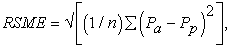(5)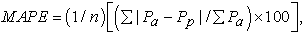(6)

If RMSE and MAPE are 0 then the model will be excellent.

Based on the sensitivity analysis, for the Mamdani model a modification on the prototype model configuration was developed using product for the AND operator, product for the implication, maximum for the aggregation, and the centroid for the defuzzification method.

4.7. FIS Model Validation

Model validation must be carried out using the input-output data that are not used for training (i.e., testing data) to evaluate the efficiency of the FIS models in predicting ultimate shear. The testing data are used in the model validation, which resulted in a total of 32 testing data for the FIS model. The FIS model prediction and target (actual) ultimate shear is used for model validation.

The performance of a FIS model can be measured to some extent by the errors on the training and testing sets, but it is often useful to investigate the model response in more detail. One option is to perform a regression analysis between the model response and the corresponding targets. Figures 10 and 11 show the results of the regression analysis between the output of the FIS model and the corresponding target for training and testing data respectively. From Figure 10 and Figure 11, R2 = 0.9969, 0.9509 for training and testing data, respectively. These values indicate an excellent agreement between the predicted and the actual values for FIS model.

### 5. Parametrical Analyses Based on the FIS Results

After the FIS model has been adequately trained, it is possible to generate new beams to study the influence of the different parameters on the shear strength. The most important conclusions of the parametrical analyses are then presented.

5.1. Influence of Concrete Compressive Strength

Figure 12 shows the effect of concrete cylinder compressive strength on ultimate shear strength of reinforced concrete beams. It can be seen that as the concrete compressive strength increases, the ultimate shear strength increases.

ACI 11-3 equation, limits the concrete compressive strength to 70MPa, leading to more conservative factor for beams with high strength concrete.

5.2. Influence of Ratio of Longitudinal Reinforcement

The influence of the ratio of longitudinal reinforcement as predicted by Fuzzy inference system is analyzed here and compared with the ACI 11-3 equation.

Figure 13 shows that the increase of amount of longitudinal reinforcement leads to increase in the ultimate shear strength. The failure shear strength is slightly increased if the amount of the longitudinal reinforcement is higher than 3.5%. However the ACI 11-3 equation do not reveals this effect for the longitudinal reinforcement and for beams with amount of longitudinal steel approximately less than 1% it may be unconservative. The FIS predicts a non-linear response of beams with the amount of longitudinal reinforcement.

5.3. Size Effect

Figure 14 shows the effect of the beam depth on the ultimate shear capacity of beams. It is obvious from this figure that the ultimate shear capacity of the concrete beam increases with the increase of the beam depth and reasonable agreement between the results of ACI-Code equation and of the FIS is achieved.

Figure 15 illustrates the relation between shear strength and beam depth for results obtains from FIS and ACI equation. This expression does not take into account the size effect on shear strength. The ACI 11-3 equation does not correlate properly with FIS results, and for beams with high effective depth it may be unconservative.

5.4. Influence of A/D Ratio

The effect of the shear span to depth ratio is depicted in Figure 16. In this figure, it can be seen that the increase of a/d, results in a decrease in the shear strength. The ultimate shear strength is reduced about 28.7% as the a/d ratio increased from 2.41 to 7. The ACI 11-3 equation do not take into consideration the influence of a/d ratio.

Figure 16. influence of shear span to depth ratio on shear strength of beams

### 6. Conclusions

This study investigates the feasibility of using fuzzy inference system to evaluate the ultimate shear strength of concrete rectangular beams without web reinforcement. The fuzzy inference system is particularly useful for evaluating systems with multitude of variables. The FIS has been proved to accurately predicting the ultimate shear strength of concrete beams without web reinforcement. FIS can be used as a reliable alternative to costly experimental testing as well as lengthy empirical calculations for predicting ultimate shear strength of concrete beams.

The ultimate shear strength of beams is found to be nonlinearly related to the ratio of longitudinal reinforcement.

The increasing of amount of longitudinal reinforced steel leads to increase the ultimate shear strength.

The influence of concrete compressive strength predicted by FIS was compared with ACI equation. ACI equation seems to be more conservative for beams with high strength concrete.

### Nomenclature

α. Shear span

a/d. Shear span to depth ratio

Aij. Input fuzzy set defined in the input space Uj

As. Area of longitudinal reinforcement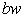. Web width

Bi. Output fuzzy set defined in the output space Vi

Ci. Constant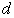. Effective depth of beam

d/bw. Total depth to width ratio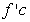. Cylinder compressive strength of concrete

n. Total number of rules

Pa. Actual values

Pp. Predicted values

Ri. Represents the ith rule

Uj. Input space

V. Ultimate shear force of beam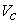. Shear strength of concrete

Vi. Output space

xj Input variables

yi. Output variable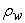. Ratio of longitudinal reinforcement area to effective web area (As/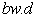)

### References

  Cladera, A., and Mari, A. R., "Shear design procedure for reinforced normal and high-strength concrete beams using artificial neural networks. Part I: beams without stirrups", Engineering Structures, 26, 2004, pp. 917-926.In article CrossRef  Collins M.P., and Kuchma, D.,"How safe are our large, lightly reinforced concrete beams, slabs and footings", ACI Struct J, 96 (4), 1999, pp. 482-490.In article  Fujita M, Sato R, Matsumoto K, Takaki Y. ,"Size effect on shear capacity of RC Beams using HSC without shear reinforcement", Proc. 6th Int Sympon Utilization of HS/HP Concrete. 2002, pp. 235-45.In article  Paratibha, A. and Yogesh, A., "Prediction of compressive strength of self compacting concrete with fuzzy logic", World Academic Science, Engineering and Technology, 77, 2011, pp. 847-854.In article  Fa-Liang, G., "A new way of predicting cement strength - fuzzy logic", Cement and Concrete Research, 27, 6, 1997, pp. 883-888.In article  Akkurt, S., Tayfurb, G., and Can, S., "Fuzzy logic model for the prediction of cement compressive strength", Cement and Concrete Research, 34, 2004, pp. 1429-1433.In article CrossRef  Demir, F., "A new way of prediction elastic modulus of normal and high strength concrete-fuzzy logic", Cement and Concrete Research, 35, 2005, pp. 1531-1538.In article CrossRef  Nataraja, M.C., Jayaram, M.A., and Ravikumar, C.N., "Prediction of early strength of concrete: a fuzzy inference system model", International Journal of Physical Sciences, Vol. 1, 2, 2006, pp. 047-056.In article  Unala, O., Demir, F., and Uygunoglua, T., "Fuzzy logic approach to predict stress–strain curves of steel fiber-reinforced concretes in compression", Building and Environment, 42, 2007, pp. 3589-3595.In article CrossRef  Topcu，I.B., and Saridemir, M., "Prediction of rubberized concrete properties using artificial neural network and fuzzy logic", Construction and Building Materials, 22, 2008, pp. 532-540.In article CrossRef  Ozcan, F., Atis, C.D., Karahan, O., Uncuoglu, U., and Tanyildizi, H., "Comparison of artificial neural network and fuzzy logic models for prediction of long-term compressive strength of silica fume concrete", Advances in Engineering Software, 40, 2009, pp. 856-863.In article CrossRef  American Concrete Institute (ACI), "Building code requirements for structural concrete" ACI 318-08, American Concrete Institute, Detroit, 2008.In article  Zadeh, L.A., "From circuit to system theory", Roc. of Institute of Ratio Eng., Vol. 50, 1962, pp. 856-865.In article  Zadeh, L.A., "Fuzzy sets", Journal of Information and Control, 8, 1965, pp. 338-353.In article CrossRef  Kirschfink, H., and Lieven, K., "Basic tools for fuzzy modeling", Liebigstrasse 20, D-52070, Aachen, Germany, 2000.In article  Fuzzy Logic Toolbox User's Guide: for Use with MATLAB, 2012.In article  Karl, H. R., Daniel, A. K., Kang, S. K., and Sina, M., "Shear database for reinforced concrete members without shear reinforcement," ACI Structural Journal, V.100, No.2, 2003, pp.240-249.In article